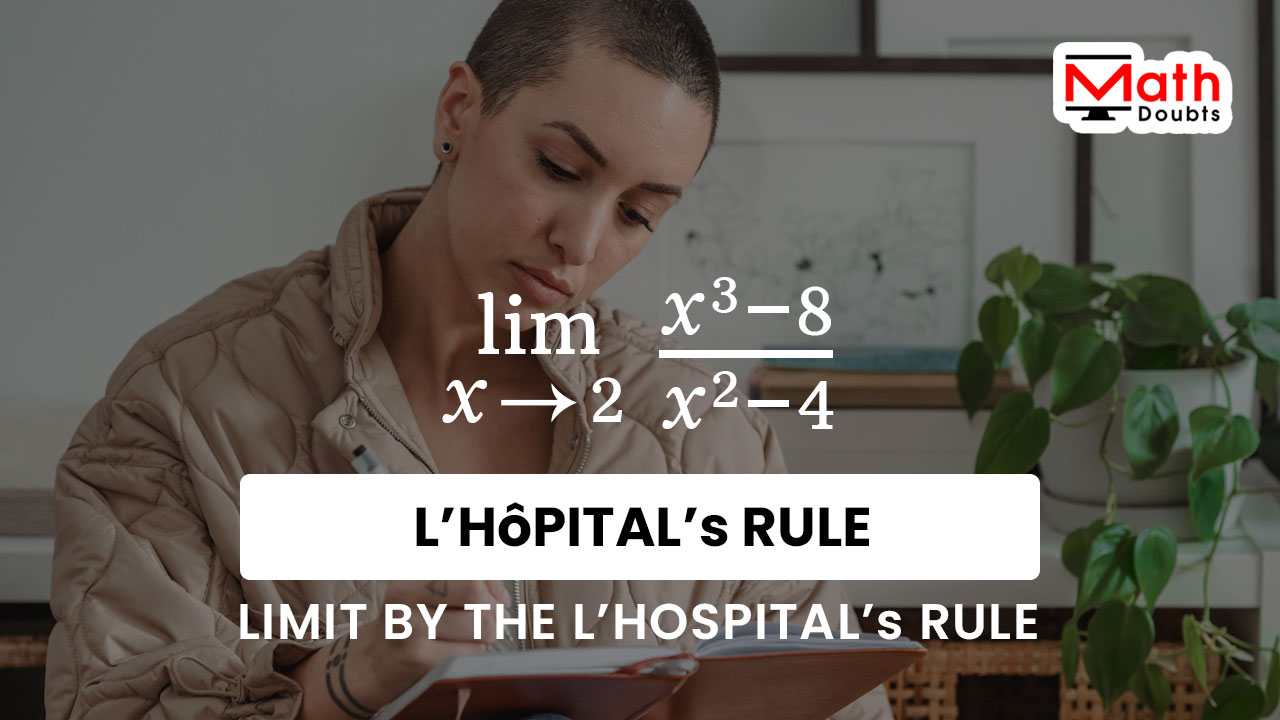# Evaluate $\displaystyle \large \lim_{x\,\to\,2}{\normalsize \dfrac{x^3-8}{x^2-4}}$ by L’Hospital’s Rule

In this limit question, the limit of cube of $x$ minus eight divided by square of $x$ minus four as the value of variable $x$ tends to two should be calculated by the l’hôpital’s rule.$\implies$ $\displaystyle \large \lim_{x\,\to\,2}{\normalsize \dfrac{x^3-8}{x^2-4}}$ $\,=\,$ $\dfrac{0}{0}$

The limit is indeterminate as per the direct substitution method. So, it is better to use the l’hospital’s rule to find the limit of $x$ cube minus $8$ divided by $x$ square minus $4$ as the variable $x$ approaches $2$.

### Apply the L’Hôpital’s rule to Limit function

According to the l’hospital’s rule, the limit of $x$ cube minus $8$ divided by $x$ square minus $4$ as $x$ tends to $2$ can be calculated by the limit of the rational function after differentiating the expression $x$ cube minus $8$ in numerator and $x$ cube minus $4$ in denominator with respect to a variable $x$.

$\implies$ $\displaystyle \large \lim_{x\,\to\,2}{\normalsize \dfrac{x^3-8}{x^2-4}}$ $\,=\,$ $\displaystyle \large \lim_{x\,\to\,2}{\normalsize \dfrac{\dfrac{d}{dx}(x^3-8)}{\dfrac{d}{dx}\big(x^2-4\big)}}$

### Differentiate the Terms in the expressions

The terms are connected by a minus sign in both numerator and denominator. So, the derivative of difference of the terms can be calculated by the subtraction rule of the derivatives.

$\,\,=\,\,$ $\displaystyle \large \lim_{x\,\to\,2}{\normalsize \dfrac{\dfrac{d}{dx}\big(x^3\big)-\dfrac{d}{dx}(8)}{\dfrac{d}{dx}\big(x^2\big)-\dfrac{d}{dx}(4)}}$

The derivatives of $x$ cube and $x$ square can be evaluated by the power rule of the differentiation. Similarly, the derivatives of the numbers $8$ and $4$ can be calculated by the derivative rule of a constant.

$\,\,=\,\,$ $\displaystyle \large \lim_{x\,\to\,2}{\normalsize \dfrac{3 \times x^{3-1}-0}{2 \times x^{2-1}-0}}$

It is time to simplify the expressions in both numerator and denominator, and it is useful to prepare the rational function in finding its limit.

$\,\,=\,\,$ $\displaystyle \large \lim_{x\,\to\,2}{\normalsize \dfrac{3 \times x^2}{2 \times x^1}}$

$\,\,=\,\,$ $\displaystyle \large \lim_{x\,\to\,2}{\normalsize \dfrac{3 \times x^2}{2 \times x}}$

$\,\,=\,\,$ $\displaystyle \large \lim_{x\,\to\,2}{\normalsize \dfrac{3 \times \cancel{x^2}}{2 \times \cancel{x}}}$

$\,\,=\,\,$ $\displaystyle \large \lim_{x\,\to\,2}{\normalsize \dfrac{3 \times x}{2 \times 1}}$

$\,\,=\,\,$ $\displaystyle \large \lim_{x\,\to\,2}{\normalsize \dfrac{3 \times x}{2}}$

### Evaluate the Limit of the Rational function

Now, substitute $x$ is equal to $2$ in the rational function to find the limit by the direct substitution.

$\,\,=\,\,$ $\dfrac{3 \times 2}{2}$

$\,\,=\,\,$ $\dfrac{3 \times \cancel{2}}{\cancel{2}}$

$\,\,=\,\,$ $3$

Latest Math Topics
Jun 26, 2023
Jun 23, 2023

Latest Math Problems
Jul 01, 2023
Jun 25, 2023
###### Math Questions

The math problems with solutions to learn how to solve a problem.

Learn solutions

Practice now

###### Math Videos

The math videos tutorials with visual graphics to learn every concept.

Watch now

###### Subscribe us

Get the latest math updates from the Math Doubts by subscribing us.